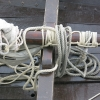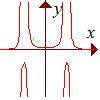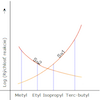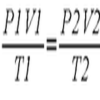# Resources tagged with: Graph sketching

Filter by: Content type:
Age range:
Challenge level:

### There are 27 results

Broad Topics > Functions and Graphs > Graph sketching### Sine Problem

##### Age 16 to 18 Challenge Level:

In this 'mesh' of sine graphs, one of the graphs is the graph of the sine function. Find the equations of the other graphs to reproduce the pattern.### Quartics

##### Age 16 to 18 Challenge Level:

Investigate the graphs of y = [1 + (x - t)^2][1 + (x + t^)2] as the parameter t varies.### Area L

##### Age 16 to 18 Challenge Level:

By sketching a graph of a continuous increasing function, can you prove a useful result about integrals?### Curve Match

##### Age 16 to 18 Challenge Level:

Which curve is which, and how would you plan a route to pass between them?### Pitchfork

##### Age 16 to 18 Challenge Level:

Plot the graph of x^y = y^x in the first quadrant and explain its properties.### A Close Match

##### Age 16 to 18 Challenge Level:

Can you massage the parameters of these curves to make them match as closely as possible?### Curve Fitter

##### Age 16 to 18 Challenge Level:

Can you fit a cubic equation to this graph?### Folium of Descartes

##### Age 16 to 18 Challenge Level:

Investigate the family of graphs given by the equation x^3+y^3=3axy for different values of the constant a.### Tangled Trig Graphs

##### Age 16 to 18 Challenge Level:

Can you work out the equations of the trig graphs I used to make my pattern?### Witch of Agnesi

##### Age 16 to 18 Challenge Level:

Sketch the members of the family of graphs given by y = a^3/(x^2+a^2) for a=1, 2 and 3.### Slide

##### Age 16 to 18 Challenge Level:

This function involves absolute values. To find the slope on the slide use different equations to define the function in different parts of its domain.### Spot the Difference

##### Age 16 to 18 Short Challenge Level:

If you plot these graphs they may look the same, but are they?### What's That Graph?

##### Age 14 to 16 Challenge Level:

Can you work out which processes are represented by the graphs?### Curvy Catalogue

##### Age 16 to 18 Challenge Level:

Make a catalogue of curves with various properties.### Guess the Function

##### Age 16 to 18 Challenge Level:

This task depends on learners sharing reasoning, listening to opinions, reflecting and pulling ideas together.### Rational Request

##### Age 16 to 18 Challenge Level:

Can you make a curve to match my friend's requirements?### Maltese Cross

##### Age 16 to 18 Challenge Level:

Sketch the graph of $xy(x^2 - y^2) = x^2 + y^2$ consisting of four curves and a single point at the origin. Convert to polar form. Describe the symmetries of the graph.### Bio Graphs

##### Age 14 to 16 Challenge Level:

What biological growth processes can you fit to these graphs?### Cocked Hat

##### Age 16 to 18 Challenge Level:

Sketch the graphs for this implicitly defined family of functions.### Polar Flower

##### Age 16 to 18 Challenge Level:

This polar equation is a quadratic. Plot the graph given by each factor to draw the flower.### Scientific Curves

##### Age 16 to 18 Challenge Level:

Can you sketch these difficult curves, which have uses in mathematical modelling?### Squareness

##### Age 16 to 18 Challenge Level:

The family of graphs of x^n + y^n =1 (for even n) includes the circle. Why do the graphs look more and more square as n increases?### Back Fitter

##### Age 14 to 16 Challenge Level:

10 graphs of experimental data are given. Can you use a spreadsheet to find algebraic graphs which match them closely, and thus discover the formulae most likely to govern the underlying processes?### Whose Line Graph Is it Anyway?

##### Age 16 to 18 Challenge Level:

Which line graph, equations and physical processes go together?### How Does Your Function Grow?

##### Age 16 to 18 Challenge Level:

Compares the size of functions f(n) for large values of n.### Reaction Types

##### Age 16 to 18 Challenge Level:

Explore the rates of growth of the sorts of simple polynomials often used in mathematical modelling.### Ideal Axes

##### Age 16 to 18 Challenge Level:

Explore how can changing the axes for a plot of an equation can lead to different shaped graphs emerging